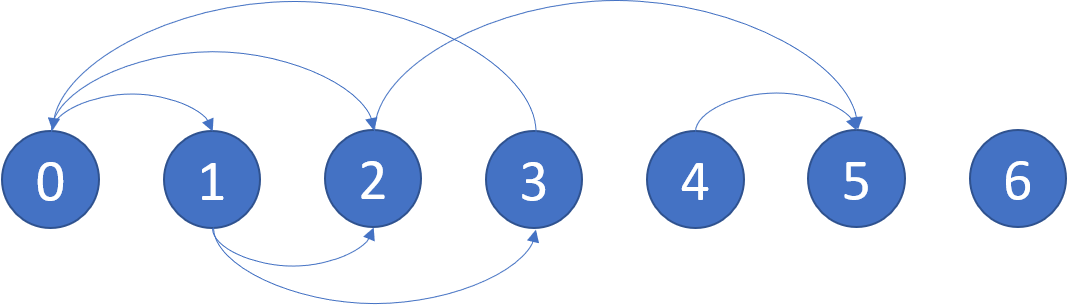802. Find Eventual Safe States
Medium
3.8K
362

There is a directed graph of `n` nodes with each node labeled from `0` to `n - 1`. The graph is represented by a 0-indexed 2D integer array `graph` where `graph[i]` is an integer array of nodes adjacent to node `i`, meaning there is an edge from node `i` to each node in `graph[i]`.

A node is a terminal node if there are no outgoing edges. A node is a safe node if every possible path starting from that node leads to a terminal node (or another safe node).

Return an array containing all the safe nodes of the graph. The answer should be sorted in ascending order.

Example 1:```Input: graph = [[1,2],[2,3],,,,[],[]]
Output: [2,4,5,6]
Explanation: The given graph is shown above.
Nodes 5 and 6 are terminal nodes as there are no outgoing edges from either of them.
Every path starting at nodes 2, 4, 5, and 6 all lead to either node 5 or 6.```

Example 2:

```Input: graph = [[1,2,3,4],[1,2],[3,4],[0,4],[]]
Output: 
Explanation:
Only node 4 is a terminal node, and every path starting at node 4 leads to node 4.
```

Constraints:

• `n == graph.length`
• `1 <= n <= 104`
• `0 <= graph[i].length <= n`
• `0 <= graph[i][j] <= n - 1`
• `graph[i]` is sorted in a strictly increasing order.
• The graph may contain self-loops.
• The number of edges in the graph will be in the range `[1, 4 * 104]`.
Accepted
129.7K
Submissions
226.4K
Acceptance Rate
57.3%

Seen this question in a real interview before?
1/4
Yes
No

Discussion (0)

Related Topics